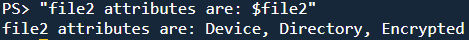This is the first of three posts that will each build on the previous post. Come back next week for the next one!

Edit - 05-May-2021 - The whole series is now live.

## So what’s an enum?

A distinct type that has a set of named constants. An enumerator is a data type that has preset values (constants). When we set a variable to an enum value it will always be one of our predefined values

## How do we create an Enum then?

we define an enum a bit like a hash table of values we want to work with, except there is no Name part, its just a set of values.

``````PS> enum Animals {
Cat
Dog
Fish
Tiger
}
``````

## How do we reference an enum once we have created one?

You can reference an enumerator with `[` and `]`

``````PS> [Animals]
``````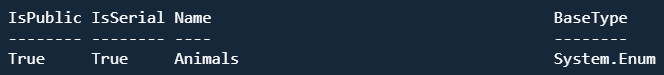``````# does that look familiar?
PS> (get-date).dayofweek
PS> (get-date).dayofweek.gettype()
``````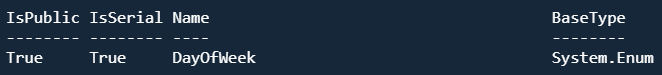We can get a specific item from the enumerator with an index value

``````PS> [Animals].GetEnumName(2)
``````## Using enums as values

Setting a variable to a value and setting a variable to an enum are similar in syntax but subtley different in what implications there are. The first results in a string variable type whereas the other results in a type that is our enum.

``````\$var1 = 'fish'
[animals]\$Var2 = [Animals]::Fish

\$Var1.gettype().fullname
\$Var2.gettype().fullname
``````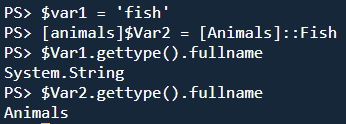## How do these two variables compare?

``````PS> (\$var1 -eq \$var2)
``````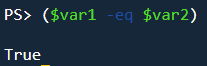We can very simply convert our Animals type variable into a string with its built-in ToString() method

``````PS> \$var2.tostring()
``````

Strange things happen when we add a second value to our [Animals] type variable though

``````PS> \$var2 += [Animals]::tiger
PS> \$var2
``````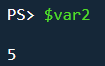The value of our variable has become the sum of the enum object values (Fish is 2 and Tige is 3 in our original declaration of the enum. However, we cant get those values back out very simply. These lines simply return `False`.

``````PS> \$var2 -eq 'fish'
PS> \$var2 -eq 'tiger'
``````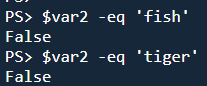Trying other comparisons is similarly doomed

``````PS> \$var2 -like 'tiger'
PS> \$var2 -eq 'fish, tiger'
PS> \$var2
``````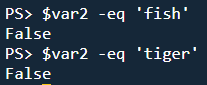The value, 5, is also useless to us as both 2+3 and 1+4 could be combinations of values that make up our current value of 5

If we want to work with more than one value in an enum then we need to declare it with the `[Flags()]` designation. Looking at the different attributes that a file can have, we could define an enum as follows

``````[Flags()] enum FileAttributes {
Archive = 1
Compressed = 2
Device = 4
Directory = 8
Encrypted = 16
Hidden = 32
}
``````

Once that is done we could define `\$file1` as `[FileAttribute]`.

``````[FileAttributes]\$file1 = [FileAttributes]::Archive
[FileAttributes]\$file1 +=[FileAttributes]::Compressed
[FileAttributes]\$file1 +=  [FileAttributes]::Device
``````

Having done this, we can see the attributes of our imaginary file with this line

``````"file1 attributes are: \$file1"
``````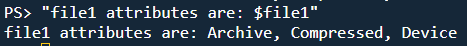Equally, we can use the sum of our selected attribute values to set our variable. Encrypted, Directory, and Device are 16 + 8 + 4 respectively, referencing our declaration above. This totals 28 so we can set our \$file2 variable as follows

``````[FileAttributes]\$file2 = [FileAttributes]28
"file2 attributes are: \$file2"
``````